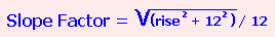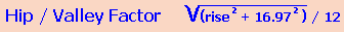# Construction Math

## Solving Unknown Lengths and Angles # I Dual Pitched Roof

What can you do with the roof pitch?##### You can gather a fair bit of knowledge from a roof pitch.
1. the roof slope (grade) in a percentage
2. the angle of the roof in degrees
3. the slope factor
4. hip valley factor
##### The grade, a slope of the roof in a percentage
To calculate the slope of the roof divide the rise by the run and multiply by 100 to get the percent value
```6  - 12 pitch
6 / 12 = 0.5 * 100 = 50 %
4 - 12 pitch
4 / 12 = 0.333 * 100 = 33.3%
```
Angle of the Roof in Degrees
##### The slope of the roof can be calculated from the roof pitch.
6/12 pitch = 0.5 then use the arctan(0.5) function and the result of this in radians = 0.4636 and in degrees 26.565

Convert degrees to radians by multiplying the degrees by π/180
where p = 3.14159265358979 and then some!
For most purposes, we are dealing with here 180/π = 57.30156 and you know something we don't need to know all this stuff! If we keep on going like this the house will never get built! Use a calculator.
##### Slope Factor

The slope factor is a pretty handy number for use in construction. With a slope factor you can calculate the square footage of a roof. To get the slope factor from the roof pitch you need to know the formula:##### Example for a 6 / 12 roof pitch:
```The rise = 6" in 12" of run
6 squared = 36
12 squared = 144
36 + 144 = 180
The square root of  180 = 13.41460786 divide this by 12 rounded to 4 digits = 1.1180
The slope factor for a 6/12 roof pitch = 1.1180
```With this factor you can calculate the square footage of the roof without having to be physically on the roof. Measure the total width of the home including the two eave's overhangs and the total length including the two gable overhangs.

#### Example:

```A home with a total width of 20' and an overall length of 45' and a 6/12 roof pitch
Multiply these two amounts to get a square footage value.
20 * 45 =  900 sq ft.  Multiply this amount by the slope factor for a 6/12 pitch.
900 * 1.1180 = 1006.2 sq ft.
```
##### Rafters, Hips and Valley's

The slope factor can also be used to calculate the length of a common rafter. All you need to know is the slope factor for your roof the length from outside wall to outside wall plus the eave overhang. And this can all be calculated standing on level ground.##### Example: calculating the rafter length from centre of the roof to the tail
```the building width from outside wall to outside wall = 20' 8"
the pitch of the roof = 6/12
the overhang of the eaves = 18"```
Split the the building width in two and add the eave measurement:
it is easier to work with by converting the feet value to inches first

`20' * 12 = 240" + 8"= 248" / 2 = 124" + plus the eave measurement 18" = 142"`
multiply this length by the slope factor of a 6 /12 roof = 1.1180 to get the rafter length
142" * 1.1180 = 158.756"
Inches + Fraction: 158 189/250
Inches + Usable Fraction: 158 3/4"
Feet + Inches:13' 3/4"

The length of the rafter is 158 3/4" or 13' 3/4"

If you are using a ridge board you will have to subtract half the thickness of the ridge board from this measurement.

A hip or valley factor is calculated with a slightly different formula.Example for a 6 /12 roof pitch
```6 squared = 36
16.97squared  = 287.9809    36 +  287.9809 = 323.9809
the square root of 323.9809 = 17.999469.......
17.999469 / 12 = 1.49995 or  rounded to 1.5000
```
The factor to calculate the length of a hip or valley rafter = 1.5000

## Roof Pitch Calculator

Roof Pitch Rise /Run

#### Results:

 Roof Slope in a Percentage % Angle of Roof in Degrees ° Rise in 12 Inches / 12 Slope Factor # Hip/Valley Factor /#